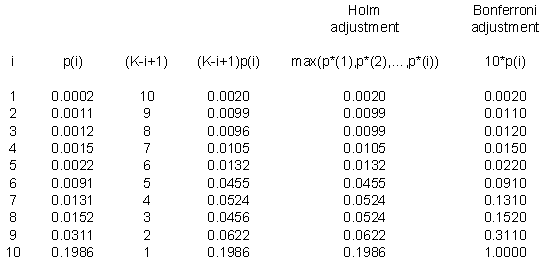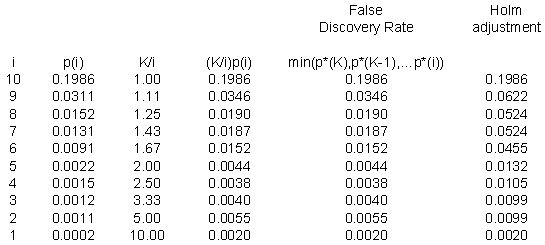## 2005-06-16

Categories: Blog post Tags:

In some research studies, you have a large and difficult to manage set of outcome measures. This is especially true in microarray experiments, where for thousands or tens of thousands of genes, you are measuring the difference in expression levels between two types of tissue. A simple p-value is worthless in this situation, because it will be swamped by thousands of other p-values.

The simplest (and least effective) adjustment to the p-values is a Bonferroni correction. It simply multiplies each p-value by the number of outcome measures. With a thousand genes, it would declare a difference anytime the unadjusted p-value was less than 0.00005. It’s impossible for a p-value to be larger than 1, so any Bonferroni adjusted p-values larger than 1 are set equal to 1.

You can get a slight improvement in Bonferroni by using a step down procedure. The Holm step-down procedure is the easiest to understand. First, sort your thousand p-values from low to high. Multiply the smallest p-value by one thousand. If that adjusted p-value is less than 0.05, then that gene shows evidence of differential expression.

That’s no different than the Bonferroni adjustment. But now that we know that the first gene is differentially expressed, we have 999 genes for which we are not sure what is going on. So multiply the next smallest p-value by 999 (not one thousand) and see if it is less than 0.05.

Multiply the third smallest p-value by 998, the fourth smallest by 997, etc. Compare each of these adjusted p-values to 0.05.

Now that is not quite the Holm step down procedure. One problem that crops up is that the adjusted p-values may not be in the same order as the unadjusted p-values. The third p-value was multiplied by 998 and the fourth by 997. If they were close enough, the order of the adjusted p-values might be reversed.

To avoid this problem, you should insure that any adjusted p-value is at least as large as any preceding adjusted p-value. If it is not make sure it is equal to the largest of the preceding p-values.

Here are the official mathematical details for the Holm step-down procedure.

Step 1. Sort the p-values from low to high:

$p_{(1)} \le p_{(2)} \le p_{(3)} \le \cdots \le p_{(K)}$

Step 2: Multiply the p-values by K, K-1, K-2,…

$p_{(1)}^*=K p_{(1)}$

$p_{(2)}^*=(K-1) p_{(2)}$

$p_{(3)}^*=(K-3) p_{(3)}$

$\vdots$

$p_{(i)}^*=(K-i+1) p_{(i)}$

$\vdots$

Step 3: Correct for any p-values out of their proper order.

$p_{(i)}^**=max_{j \le i}p_{(j)^*}$

Here’s a small example. Suppose I have ten p-values: 0.0002, 0.0011, 0.0012, 0.0015, 0.0022, 0.0091, 0.0131, 0.0152, 0.0311, and 0.1986. These are impressively small, even after accounting for the fact that we have ten of them.Notice that the first part of the Holm adjustment (the fourth column) leaves a few of the adjusted p-values out of order. The third p-value is smaller than the second and the eighth p-value is smaller than the seventh. When you take the maximum of any preceding p-values (the fifth column), that puts a proper order to the adjusted p-values.

The last column is the Bonferroni adjustment, which simply multiplies each p-value by 10. Notice that there is a slight difference, since the Bonferroni adjusted p-value for the sixth row is not statistically significant, though it is significant using the Holm step-down procedure.

A better approach than either Bonferroni or the Holm step-down procedure is to define a false discovery rate and control that value directly rather than the p-value.

To understand the false discovery rate better, suppose that there are K genes, that a procedure declared R genes to be statistically significant and that V of these genes are false positives. Both Bonferroni and the Holm step-down procedure insure that P[V>0] is no more than alpha. In a microarray experiment, this is a rather stringent standard. Most researchers will tolerate a handful of false positive genes. A microarray experiment is often an exploratory study. Most of the time, these researchers follow up on any positive findings with additional testing anyway. So a small number of false positives is tolerable. They just don’t want the number of false positives to dominate the list of statistically significant genes.

Suppose you ran an microarray experiment.with 10,000 genes and the largest p-value before any adjustment was 0.05. This is like hitting the lottery jackpot, since every gene is statistically significant. Testing at an unadjusted alpha level of 0.05 tells you that the expected number of false positives is 500 (5% of 10,000). That’s a tolerable number among 10,000 positive results.

Let’s suppose though that only half of your genes (5,000) are statistically significant at an unadjusted alpha level of 0.05. Now the number of false positives represents 10% of the significant genes. Are you starting to get a bit worried? Maybe not, but now suppose a quarter of your genes (2,500) are statistically significant at an unadjusted alpha level of 0.05. The expected number of false positives is still 500, but now that represents 20% of the genes, which might make you a bit uncomfortable. Suppose that 10% of your genes (1,000) are statistically significant at an alpha level of 0.05. Now the false positives are going to be half of the positive genes.

So perhaps you should apply a stricter standard when fewer of your genes are statistically significant. In the last situation, it might make sense to test at an alpha level that is one-tenth as large (0.005), because then the expected number of false positives (50) is only 5% of the total number of genes again.

So to control the false discovery rate, don’t bother adjusting the largest p-value, adjust the p-value halfway through the list by doubling it, adjust the p-value three quarters of the way towards the smallest p-value by quadrupling it, adjust the p-value nine-tenths of the way down by multiplying by 10, etc.

Notice that the false discovery rate proceeds in the opposite order from the Holm adjustment. It looks at the largest p-value first. You need to insure consistency in the ordering of the false discovery rates, but here you make sure that the second largest false discovery rate is not larger than the largest, that the third largest false discovery rate is not larger than the previous two, etc..

The formal mathematical definition is

Step 1. Sort the p-values from high to low:

$p_{(K)} \ge p_{(K-1)} \ge p_{(K-2)} \ge \cdots \ge p_{(1)}$

Step 2: Multiply the p-values by 1, K/(K-1), K/(K-2),…

$p_{(K)}^*=p_{(K)}$

$p_{(K-1)}^*=p_{(K-1)}\frac{K}{(K-1)}$

$p_{(K-2)}^*=p_{(K-2)}\frac{K}{(K-2)}$

$\vdots$

$p_{(K-i)}^*=p_{(K-i)}\frac{K}{(K-i)}$

$\vdots$

$p_{(1)}^*=p_{(i)} K$

Step 3: Correct for any p-values out of their proper order.

$p_{(K-i)}^**=min_{j \le i}p_{(K-i)}^*$

Here’s the same example using the ten p-values discussed above. It seems a bit silly, perhaps to apply the false discovery rate to such a small number of p-values, but it does help to illustrate the calculations.The p-values for the Holm adjustment are listed at the end for comparison.

The false discovery rate is not a p-value. Instead, think of it as the proportion of false positive results that you are willing to tolerate among the results that are flagged.

To understand this better, define R as the number of genes that are flagged as differentially expressed by a procedure (note that I did not say the number of genes that are statistically significant). Let V represent the number of false positives among those R genes. I’m using the notation which appears in the book

• Amaratunga and Cabrerea, Exploration and Analysis of DNA Microarray and Protein Array Data.

The Bonferroni approach (and the Holm adjustment) control the familywise error rate, which is defined as

$P[V > 0]$

This is saying that even a single false positive is intolerable and you must control this scrupulously. For a microarray experiment, this may be too strict. Instead you should focus on the ratio of false positives, V/R. A formal mathematical definition here is a bit tricky, because we can’t allow even a small probability that the denominator is zero. It turns out that the false discovery rate is equal to

$E\big[\frac{V}{R}|R > 0\big]P[R > 0]$

Once you let go of the concepts of p-values, then you can drop the dogmatic insistence on an alpha level of 0.05 as well.

How many false positives can you tolerate in a sample of genes that are flagged as differentially expressed? In many situations, I suspect that your tolerance would be fairly high maybe 10% or 20%. Amaratunga and Cabrera suggest that in some situations, a false discovery rate of 50% might still be tolerable.

Think carefully about this choice. What is the cost of a false positive? In a microarray experiment, it means tracking down a lead that ends up being a dead end. This is not a trivial cost, but your perspective here is quite different than testing a new drug, for example. There, a false positive means allowing an ineffective drug onto the market.

You can find an earlier version of this page on my original website.